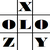anything in Excel...

It's Excel-o-lozy...

### Formula to convert date from Raw text format to valid date format?

www.Excelolozy.com - Converting YYYYMMDD to DDMMYYYY i.e. the Date from Raw text format to recognisable Date format... It is always time consuming, and tough.

e.g. To convert date format from YYYYMMDD to DDMMYYYY a little long combination of 2-3 formulas is required.

To convert raw date format directly to system recognisable date format, following formula works wonders :
formula is "ConvertDate"

=ConvertDate(Ref,OldFormat)

where...

Ref is Reference of the cell where Raw data is entered.
and OldFormat is the Format in which the raw data exists.

an Example is hereunder...

if Raw date in Cell A1 is 20121013

Now 2 options are there...

1) If it is written in YYYYMMDD format...

then formula should be...

=ConvertDate(A1,"YYYYMMDD")

or

=ConvertDate(A1,)

even this will work...

because default format is YYYYMMDD...

Result would be "13-Oct-2012".

2) If it is written in DDMMYYYY format...

then formula should be...

=ConvertDate(A1,"DDMMYYYY")

and Result would be "20-Dec-1013".

Amazing... isn't it???

Now what this "ConvertDate" formula is all about? How to use it? How to integrate it with Excel...